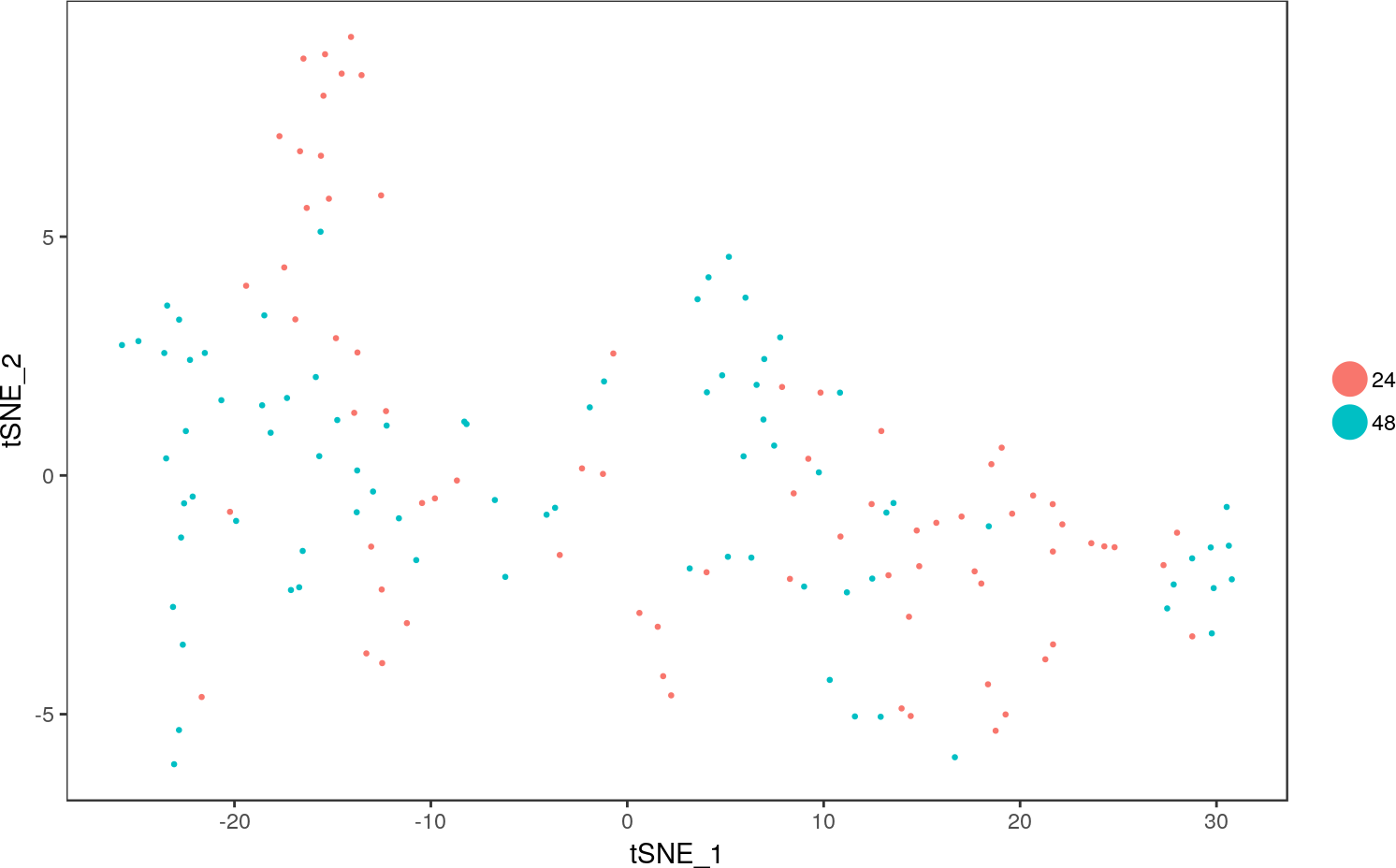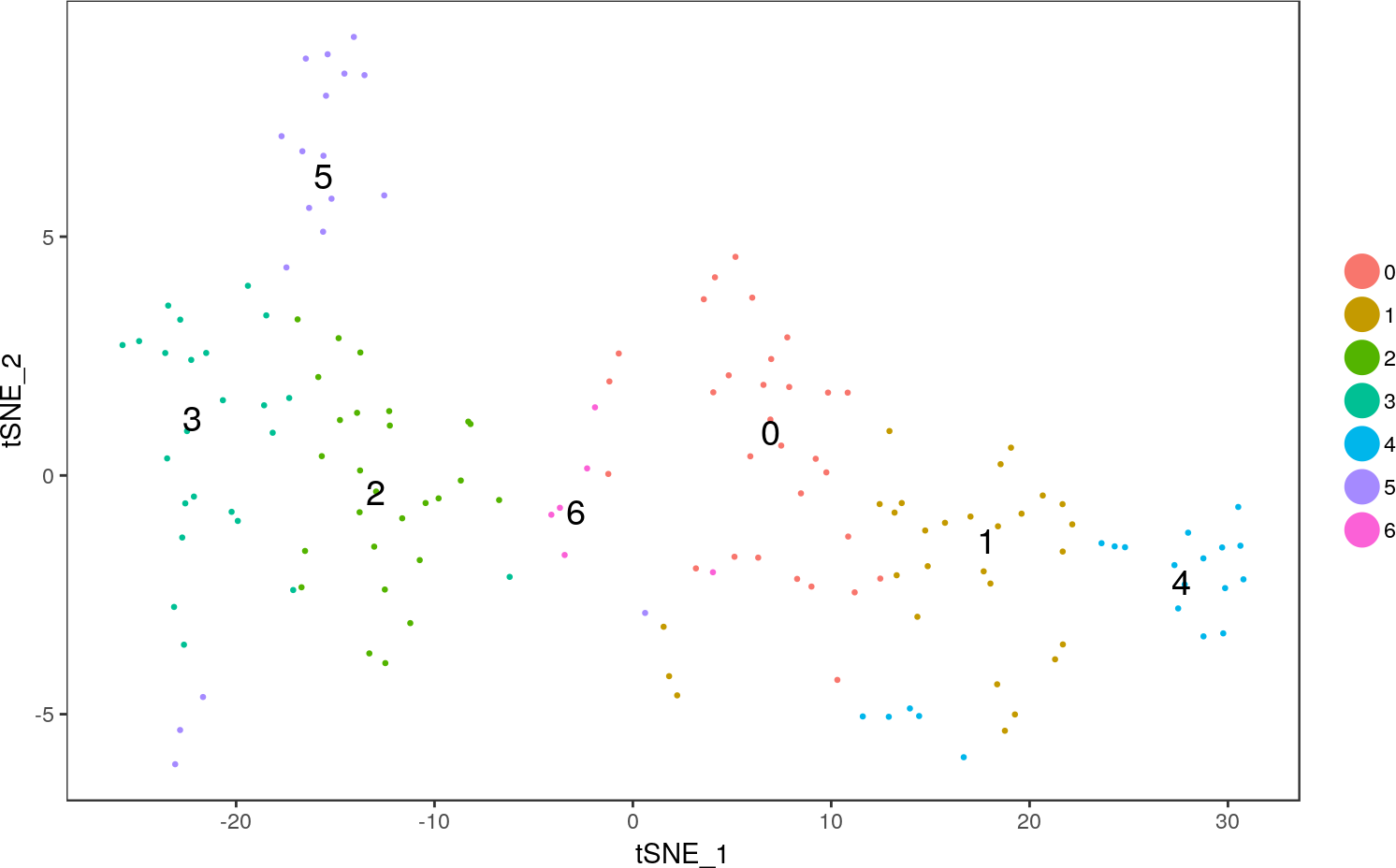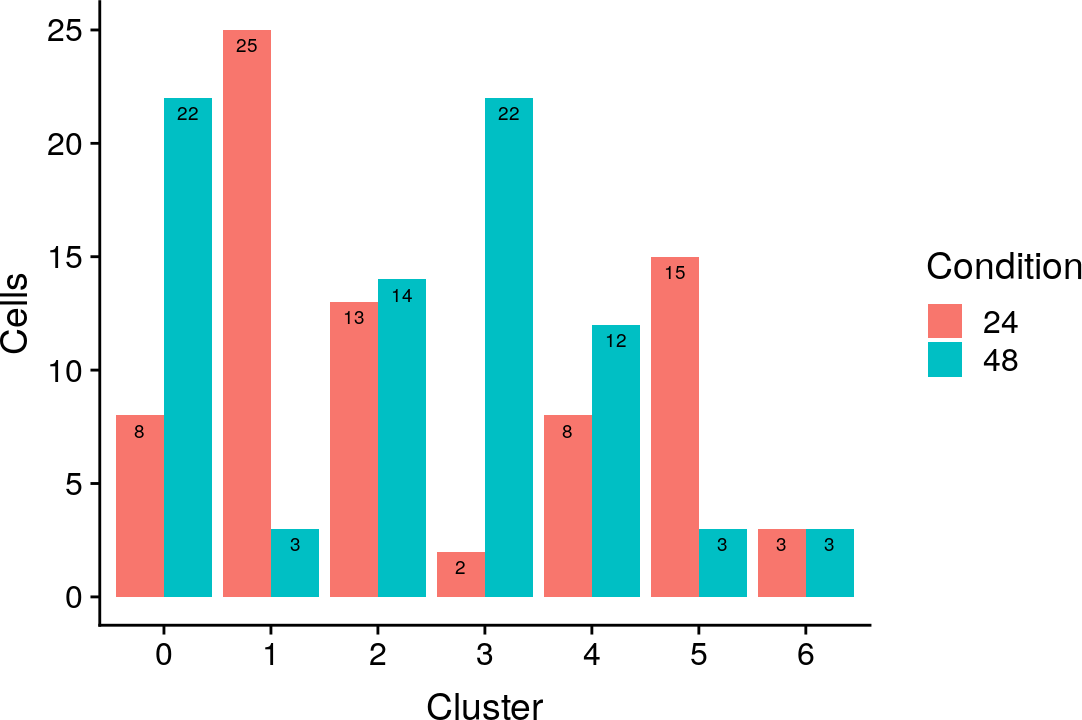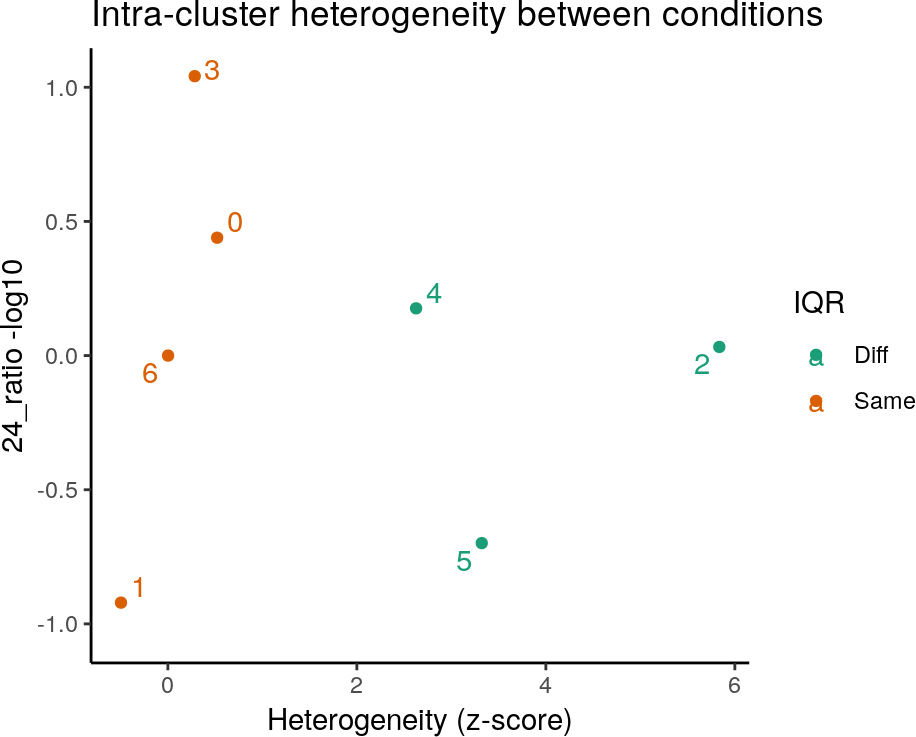# 1 Introduction

When analysing scRNA-seq data it is not uncommon to perform comparison between two given conditions present in the data. For each cell type identified, these conditions may have different profiles. The condcomp package aims to assist in the characterization of those differences in an easy and direct way.

For this vignette we will be use the HSMM data from monocle which is available through the HSMMSingleCell package. The data pertains information on an experiment where primary human skeletal muscle myoblasts (HSMM) were expanded under high mitogen conditions (GM) and then differentiated by switching to low-mitogen media (DM). RNA-Seq libraries were sequenced from each of several hundred cells taken over a time-course (0, 24, 48, 72 hours) of serum-induced differentiation.

We will use the Seurat package in order to perform the analysis of the data.

# 2 Installation

The package can be installed using the chunk below.

BiocManager::install("condcomp")

# 3 Using the package

Firstly we load the data and encapsulate it in a Seurat object. We will use only only times 24 and 48 in our example as those will be the two condtions to be analysed.

library(condcomp)
library(monocle)
library(HSMMSingleCell)
library(Seurat)

# Encapsulate data in a Seurat object
hsmm <- exportCDS(hsmm, export_to = "Seurat")
# Set ident to 'Hours'
hsmm <- SetAllIdent(hsmm, id = "Hours")
# Subset the Seurat object to have cells only from 24 and 48 hours
hsmm <- SubsetData(hsmm, ident.use = c("24", "48"))
# Stores this ident as a 'Condition' column in 'meta.data'
hsmm <- StashIdent(hsmm, save.name = "Condition")

Next we will find the highly variable genes for this data and use them to build the PCA space. Finally, we cluster our data using the Seurat function FindClusters and project the data onto the t-SNE space for visualisation.

The resolution parameter of FindCluster was set from the default value of one to two, in order to increase the amount of clusters given by the algorithm. The perplexity parameter was reduced from the default value of 30 to 15, as this data does not have many data points.

hsmm <- FindVariableGenes(hsmm, do.plot = FALSE)
hsmm <- RunPCA(hsmm)

hsmm <- FindClusters(hsmm, reduction.type = "pca", dims.use = 1:5,
resolution = 2)
hsmm <- StashIdent(hsmm, save.name = "Cluster")
hsmm <- RunTSNE(hsmm, reduction.use = "pca", dims.use = 1:5, do.fast = TRUE,
perplexity = 15)
TSNEPlot(hsmm, group.by = "Condition", do.return = TRUE, pt.size = 0.5)TSNEPlot(hsmm, do.return = TRUE, pt.size = 0.5, do.label = TRUE,
label.size = 5)The bar plot below shows the amount of cells in each condition grouped by cluster.

hsmm <- SetAllIdent(hsmm, "Cluster")
counts <- as.data.frame(table(hsmm@meta.data$Condition, hsmm@ident)) names(counts) <- c("Condition", "Cluster", "Cells") ggplot(data = counts, aes(x = Cluster, y = Cells, fill = Condition)) + geom_bar(stat="identity", position = position_dodge()) + geom_text(aes(label = Cells), vjust = 1.6, color = "black", position = position_dodge(0.9), size = 2.5)Having the clustering set up, we can now use condcomp in order to gain insight about the heterogenity between conditions for each cluster. Ideally, these clusteres would be annotated with cell types, although that does not diminish the usefulness of the analysis provided by condcomp. For a description of each column in the resulting data frame, please refer to the manual page for condcomp. # Computes the euclidean distance matrix dmatrix <- dist( GetDimReduction(hsmm, reduction.type = "pca", slot = "cell.embeddings"), method = "euclidean") dmatrix <- as.matrix(dmatrix) hsmm <- SetAllIdent(hsmm, "Cluster") ccomp <- condcomp(hsmm@ident, hsmm@meta.data$Condition, dmatrix, n = 1000)
# It is pertinent to compute the adjusted p-value, given the computation method
# (see the manual for 'condcomp')
ccomp$pval_adj <- p.adjust(ccomp$pval, method = "bonferroni")
knitr::kable(ccomp)
24_perc 48_perc 24_ratio 48_ratio true_sil zscore pval iqr pval_adj
0 0.2666667 0.7333333 0.3636364 2.750000 0.0241758 0.5215740 0.271 Same 1.000
1 0.8928571 0.1071429 8.3333333 0.120000 -0.0328375 -0.4959992 0.652 Same 1.000
2 0.4814815 0.5185185 0.9285714 1.076923 0.0862150 5.8358145 0.000 Diff 0.000
3 0.0833333 0.9166667 0.0909091 11.000000 0.0115130 0.2843489 0.381 Same 1.000
4 0.4000000 0.6000000 0.6666667 1.500000 0.0732389 2.6268979 0.011 Diff 0.077
5 0.8333333 0.1666667 5.0000000 0.200000 0.2541525 3.3216138 0.001 Diff 0.007
6 0.5000000 0.5000000 1.0000000 1.000000 0.0052120 0.0024497 0.307 Same 1.000

Next we plot the results of the analysis. We can see that group 6, despite having a 1:1 ratio between conditions, has a low Z-score, which indicates a low heterogeneity within said group despite the seemingly heterogeneity stemming from the condition ratio. In contrast, group 2, which has a near 1:1 ratio between conditions, has a high Z-score, reinforcing the apparent heterogeneity that stems from the condition ratio.

Groups which one of the conditions is more predominant tend to have lower Z-scores. Groups which this condition predominance is observed but have a considerable high Z-score might be worth investigating. This could indicate a poor performance on clustering or indeed an interesting group that must be more meticulously analysed.

We can see that the IQR based approach is indicating ‘Same’ for some groups. Although that information should not be considered alone (see the man page of condcomp for details regarding the computation of the IQR): it is one of the indicators of heterogeneity. The other indicators being: the ratio between conditions, the Z-score, and the p-value. The last is present in the previous table but not in the plot below. In this example, it is evident that all the groups with low Z-score also have a value of ‘Same’ for IQR.

For this data some p-values were exactly or near zero (even after the correction), which indicate a considerable heterogeneity for those groups. Conversely a high p-value indicates a low heterogeneity.

Note that the parameter n should be set accordingly. The greater this value, the more reliable are the results at the cost of an increase in execution time. In this vignette we used the dafault value of 1,000 but, depending on the number of objects (cells) in the dataset, a much greater value should be used. If unsure, setting n = 10000 should be a fairly reasonable value for typical single cell datasets.

condcompPlot(ccomp, main = "Intra-cluster heterogeneity between conditions")The main objective of this plot is to assist with the detection of heterogeneous groups with respect to the conditions. These groups can be sources of valuable information in differential analysis and group profiling.

This plot is even more powerful if the data is annotated. For instance, we could perform condcomp on identified cell types instead.